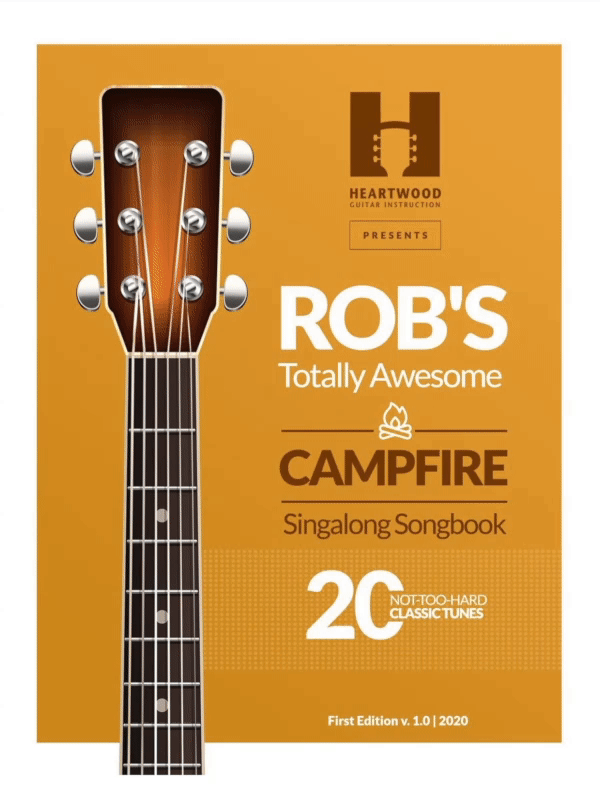# 20 CLASSIC SONGS your friends will love

Beatles - Petty - Johnny Cash - Eagles - John Denver - DylanThis chart will look wacky unless you

Give a Little Bit

Supertramp

Chords in parentheses are anticipated.

Intro:  / - - - (A7) /

/ (D) - - (A7) / (D) - - (G) /

/ (A7) - - (G) / (A7) - - (G) /

/ (D) - - (A7) / (D) - - (G) /

/ (A7) - - (G) / (A7) - - (G) /

/ (D) - - (A7) /        / (D) - - (G) /

Give a little bit        give a little bit of your

/ (A7) - - (G) / (A7) - - (G) /

love to me

/ (D) - - (A7) /        / (D) - - (G) /

I'll give a little bit        I'll give a little bit of my

/ (A7) - - (G) / (A7) - - (G) /

love to you

Bm                              / Esus4 - (E) - /

There's so much that we    need to share,

G              / A13sus4 - (A7) - / A13sus4 - (A7(no3)) - /

So send a smile and show you care                Alright

/ (D) - - (A7) /        / (D) - - (G) /

I'll give a little bit,      I'll give a little bit of my

/ (A7) - - (G) / (A7) - - (G) /

life for you,

/ (D) - - (A7) /        / (D) - - (G) /

So give a little bit,       oh, give a little bit of your

/ (A7) - - (G) / (A7) - - (G) /

time to me,

Bm                       / Esus4 - (E) - /

See the man with the lonely eyes

G                 / A13sus4 - A7 - / A13sus4 - A7(no3) - /

Oh, take his hand, you'll be surprised

Bridge: / F#m - - - / Bm - - - /

/ F#m - - - / Bm - - - /

/ F#m - - - / G - - - / Cadd9 - (G) - /

/ A7 - A13sus4 - / A7 - A13(no3) - / x3

/ (D) - - (A7) /        / (D) - - (G) /

Give a little bit,       oh, give a little bit of your

/ (A7) - - (G) / (A7) - - (G) /

love to me,

/ (D) - - (A7) /        / (D) - - (G) /

I'll give a little bit,      I'll give a little bit of my

/ (A7) - - (G) / (A7) - - (G) /

life for you,

Bm                           / Esus4 - (E) - /

Now's the time that we    need to share,

G                    / Cadd9 - (G) - /

So find yourself, we're on our way back home,

/ A7 - A13sus4 - / A7 - A13(no3) - /

Going home

/ A7 - A13sus4 - / A7 - A13(no3) - /

don’t you need, don't you need to feel at home?

/ A7 - A13sus4 - / A7 - A13(no3) - /

Oh yeah    we gotta sing

Outro:

/ D - (G/D) - / (A/D) (G/D) (D) - / C/D - - - / G/D - (D) - / x4

/ C/D - - - / A/D - (D) - / x2

Come along do   For a long ride

/ Cadd9 - G/B - / A - /

Come a long way  oh  sing it tonight

/ D - (G/D) - / (A/D) (G/D) (D) (hold) /

End on D

This file is the author's own work and represents his interpretation of this song. It's intended solely for private study, scholarship or research.